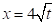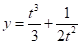Chapter 10, Problem 41RE

Chapter
Section
Textbook Problem

Find the area of the surface obtained by rotating the given curve about the x-axis.41.,, 1 ≤ t ≤ 4

To determine

To find: The area of the surface obtaining by rotating the curve x=4t and y=t33+12t2 for 1t4 about x-axis.

Explanation

Given:

The parametric equation for the variable x is as follows.

x=4t (1)

The parametric equation for the variable y is as follows.

y=t33+12t2 (2)

Calculation:

Differentiate the equation (1) with respect to t .

dxdt=4×12(t12)

dxdt=2(t12)

dxdt=2t (3)

Differentiate the equation (2) with respect to t .

dydt=3t2t3

dydt=t21t3 (4)

Surface area is determined for the limits of 1 to 4 .

Calculate the surface area the curve rotating about x axis using the formula.

S=142πy(dxdt)2+(dydt)2dt (5)

Substitute the expressions from equation (2), (3) and (4) into equation (5).

S=142πy(dxdt)2+(dydt)2dt=142π(t33+12t2)(2t)2+(t21t3)2dt=142π(t33+12t2)4t+t4+1t6(2t2×1t3)dt

S=142π(t33+12t2)4t+t

Still sussing out bartleby?

Check out a sample textbook solution.

See a sample solution

The Solution to Your Study Problems

Bartleby provides explanations to thousands of textbook problems written by our experts, many with advanced degrees!

Get Started

Sketch the graphs of the equations in Exercises 512. 2y+x=1

Finite Mathematics and Applied Calculus (MindTap Course List)

In Exercises 107-120, factor each expression completely. 117. 9x2 16y2

Applied Calculus for the Managerial, Life, and Social Sciences: A Brief Approach

In Exercises 9-12, find the domain of the function. f(x)=1x1

Calculus: An Applied Approach (MindTap Course List)

Find the derivatives of the functions in Problems 1-34. 19.

Mathematical Applications for the Management, Life, and Social Sciences

Find all degree solutions. tan23=3

Trigonometry (MindTap Course List)

Sometimes, Always, or Never: The sum of two elementary functions is an elementary function.

Study Guide for Stewart's Single Variable Calculus: Early Transcendentals, 8th

The quadratic approximation for at a = 1 is:

Study Guide for Stewart's Multivariable Calculus, 8th